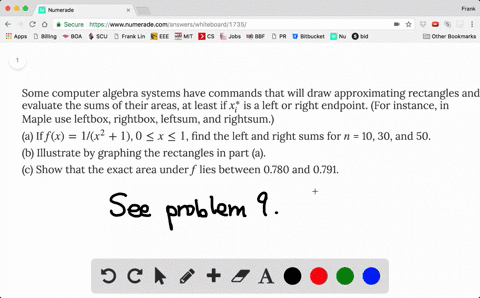### Some computer algebra systems have commands that …

00:15
FL
Problem 10

With a programmable calculator (or a computer), it is possible to evaluate the expressions for the sums of areas of approximating rectangles, even for large values of $n$, using looping. (On a TI use the Is> command or a For-EndFor loop, on a Casio use Isz, on an HP or in BASIC use a FOR-NEXT loop.) Compute the sum of the areas of approximating rectangles using equal subintervals and right endpoints for $n$ = 10, 30, 50, and 100. Then guess the value of the exact area.

The region under $y = \cos x$ from 0 to $\pi/2$

$R_{10}=0.919403, R_{30}=0.973592, R_{50}=0.98421$
$R_{100}=0.992125, \quad$ exact value guess: 1

## Discussion

You must be signed in to discuss.

## Video Transcript

So this is a computer problem. I leave that detail for you and to know how to set it up. I explain in problem night off this section. So if you want more information, please take a look at that.